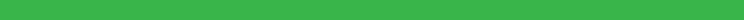SEARCH HOMEMath Central Quandaries & QueriesQuestion from juliana, a student: how many times can i walk around 2 acres of land to equal 1 mile?Juliana,

An acre is a unit of area and it can be in any shape.An acre is 43560 square feet in an acres so 2 acres is 87120 square feet. If it is in the shape of a square then each side is √87120 = 295.16 feet so once around is 1180.64 feet. there are 5280 feet on a mile so approximately four and a half times around is a mile.If the two acre plot is two square acres placed side by side then the perimeter is 1252.26 feet and approximately four and a quarter times around is a mile.If the two acre plot is 50 feet by 1742.4 feet then the perimeter is 3584.8 feet and approximately one and a half times around is a mile.

PennyMath Central is supported by the University of Regina and The Pacific Institute for the Mathematical Sciences.